### Seeing is believing!

Before you order, simply sign up for a free user account and in seconds you'll be experiencing the best in CFA exam preparation.

##### Subject 6. Marginal Revenue, Marginal Cost and Profit Maximization
Revenue is the income generated from the sale of output in product markets.

• Total revenue (TR) is the sum of individual units sold multiplied by their respective prices: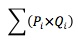• Average revenue (AR) =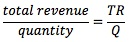• Marginal revenue (MR) is the change in revenue from selling one extra unit of output: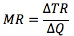In a perfectly competitive market, each firm is a price taker. Since each unit of output sold by a price taker is sold at the market price, the MR for each unit is also equal to the market price, i.e., P = MR.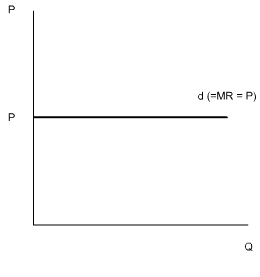Under imperfect competition, a firm's marginal revenue is always less than the price of its good. Why? As the firm reduces price in order to expand output and sales, there will be two conflicting influences on total revenue.

• The increase in sales due to the lower price will, by itself, add to the revenue of the monopoly.
• The price reduction, however, also applies to units that would otherwise have been sold at a higher price. This factor itself will cause a reduction in total revenue.

These two conflicting forces will result in marginal revenue - the change in total revenue - that is less than the sales price of the additional units. Thus, the marginal revenue curve of the firm will always lie below the firm's demand curve, which is also the market's demand curve.TR is maximized when MR = 0.

Total, Average, Marginal, Fixed, and Variable Costs

To produce more output in the short run, the firm must employ more variable inputs, which means that it must increase its costs. In the short run, a firm's total costs (TC) can be broken down into two categories: fixed costs and variable costs (TC = TFC + TVC). Which costs are fixed and which costs are variable depends on the time horizon being dealt with. For a short time horizon, most costs are fixed. For a long time horizon, all costs are variable.

• Total Fixed Cost. The sum of the costs that do not vary with output. They will be incurred as long as a firm continues in business and the assets have alternative uses. Examples of fixed costs include rent, property taxes and insurance premiums.

• Average Fixed Cost. Total fixed cost divided by the number of units produced. It always declines as output increases.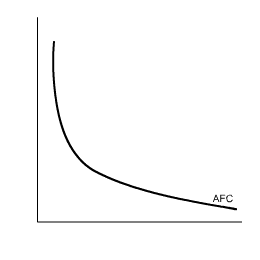• Total Variable Cost. The sum of those costs that rise as output increases. Total variable costs are zero if output is zero. Examples are wages paid to workers and payments for raw materials.

• Average Variable Cost. The total variable cost divided by the number of units produced.

• Average Total Cost. Total cost divided by the number of units produced. It is sometimes called per unit cost.

ATC is high at low levels of output, decreases as output increases (since fixed costs are spread across more units), and then increases as the firm's maximum capacity is approached (since marginal costs increase).

• Marginal Cost. The change in total cost required to produce an additional unit of output.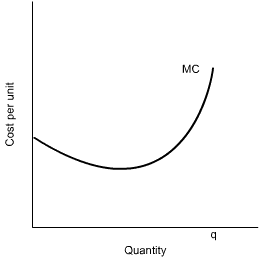The law of diminishing returns implies that the marginal costs of producing each additional unit will increase by increasing amounts. Initially, as output expands, the cost of producing each additional unit of output falls, but then begins to rise as the firm approaches its maximum capacity (e.g., too many workers, congested production lines).Over the output range with increasing marginal returns, marginal cost falls as output increases. Once a firm confronts diminishing returns, larger and larger additions of the variable factor are required to expand output by one unit. This will cause marginal cost (MC) to rise. As MC continues to increase, eventually it will exceed average total cost. Until that point, MC is below ATC (per unit cost), bringing ATC down. When MC is greater than ATC, the additional units cost more than the average, and ATC must increase. A U-shaped short-run average total cost curve results.

The vertical distance between the AVC and ATC curves measures AFC. The vertical distance between the AVC and ATC curves gets smaller as output increases because AFC decrease as output expands.

Profit Maximization

Maximum economic profit requires that (1) marginal revenue (MR) equals marginal cost (MC) and (2) MC not be falling with output.

• For firms under conditions of perfect competition, price is identical to marginal revenue (MR).
• For firms under conditions of imperfect competition, marginal revenue (MR) is less than price.

Learning Outcome Statements

d. describe the phenomenon of diminishing marginal returns;

CFA® 2022 Level I Curriculum, , Volume 2, Reading 8

User Comment
achu Rem: Var cost increase shifts all cost curves, Fixed cose incr. doesn't affect the MC curve since there is no 'per unit' cost of add'l FC.
hannovanwyk "Rem: Var cost increase shifts all cost curves"

I dont agree with achu that if VC increases FC necessarily increases. I think it is as in the notes,

"An increase in a variable cost shifts the total cost (TC), average total cost (ATC), and marginal cost (MC) curves upward." - nowhere is FC mentioned.
sgossett86 I had this in school. The only tough thing I'm having here is why marginal revenue naturally declines faster than price. Is marginal revenue equal to marginal profit? Otherwise why would decreasing the price decrease the marginal revenue faster..
michaeloa3 ^because the price change effects all goods sold, not just the addition unit.

Example: Output starts at 300 units at \$12 each. TR = \$3600, MR/unit = \$12. Due to an assortment of factors, current output is now 400 units at \$10 each, so TR = \$4000. While this example isn't perfect, it illustrates how MR drops declines with a production increase. MR for the addition 100 units is \$4000-\$3600 = \$400. MR/unit = \$1 for those additional 100 units as opposed to \$12 for the first 300. Why is this? Because the original 300 units are also sold for \$10, not just the additional 100 units.

Say that selling at \$8/unit yields another 50 units of demand. You sell 500 units for \$8, so TR = \$4,000. While price has only dropped \$4/unit, the MR/unit has dropped from \$12/unit to \$0/unit.
choas69 can someone explain (2) MC not falling with output???
choas69 for profit maximization a firm should operate at the level of output for which MR=MC, provided MC is not falling at that level.

so basically u have to reduce MC to the point in which it cant be reduced anymore and equals to MR.